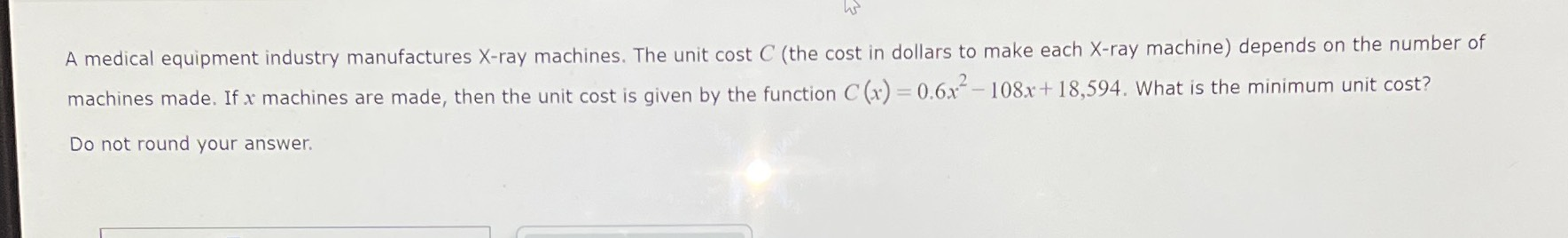### ¿Todavía tienes preguntas de matemáticas?

Pregunte a nuestros tutores expertos
Algebra
PreguntaA medical equipment industry manufactures $$X$$ -ray machines. The unit cost $$C$$ (the cost in dollars to make each $$X$$ -ray machine) depends on the number of machines made. If $$x$$ machines are made, then the unit cost is given by the function $$C ( x ) = 0.6 x ^ { 2 } - 108 x + 18,594$$ . What is the minimum unit cost? Do not round your answer.